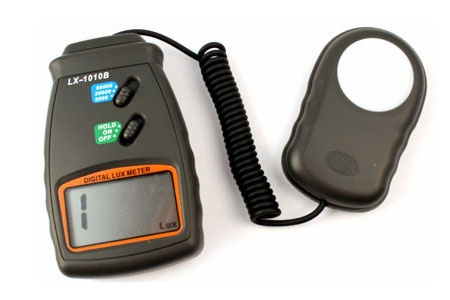Difference Between Lux and Lumens

What are Lumens?

Lumen is a unit of light measurement otherwise known as luminous flux.

We use lumens to compare the total amount of light output from a light emitter. However, lumens isn't the be-all and end-all. In fact, lumens will only tell you one part of the picture because when it comes to producing a great beam pattern, it doesn't give you enough information to tell you how the light output is used. A comparable analogy of lumens is an automobile's total brake horsepower (BHP).

To measure lumens, we use a highly specialized light integrating sphere.What is Lux?

Lux is a unit of light measurement taking area into account. In other words, light intensity.

We use lux to measure the amount of light output in a given area, where one lux is equal to one lumen per square meter. Lux is a great measurement for determining what we see as the brightness of a beam. If the light output is concentrated over a smaller area, we see this as very bright. If the light output is spread over a larger area, we see this as very weak. We normally use mirrors, reflectors, and optics to control the path of light and create the desired beam pattern. Lux also determines the magnitude of light intensity travelling over distances. A light that is configured for high lux output will travel farther but will have a smaller footprint of light (e.g. lighthouse spotlight), and a low lux level will be configured to travel shorter distances but have a larger footprint (e.g. decorative down lighting or ambient lighting).

To measure lux at a specific location, we use a device called a lux meter.Beam Angle (FWHM)

What is it?: Beam angle is the angle of which a light is emitted. More specifically, it is the Full Width at Half Maximum.

Since there is no real way to measure the 'edge' of light we measure the beam angle from where the light is at 50% intensity (FWHM). By being able to measure where 50% light intensity ends, it gives us the majority of where the light is used thus representing the beam angle. Smaller beam angles will have an intense hot spot, where as larger beam angles will have a lesser intensity (due to a larger area). Take a look at our beam shots and see if you can gauge where 50% intensity ends, giving you the beam angle.Watts

What is it?: Watt is a unit of power consumed.

The more power a light emitter consumes, the more lumens it should produce. However, the relationship is non-linear and we can use the numbers from wattage and lumens to measure the efficiency of a light emitter. LEDs have far outpaced the industry as the most efficient light emitter and can produce more light than older halogen lights. One thing to note is the more power is consumed, the more heat is produced, which is wasted energy.